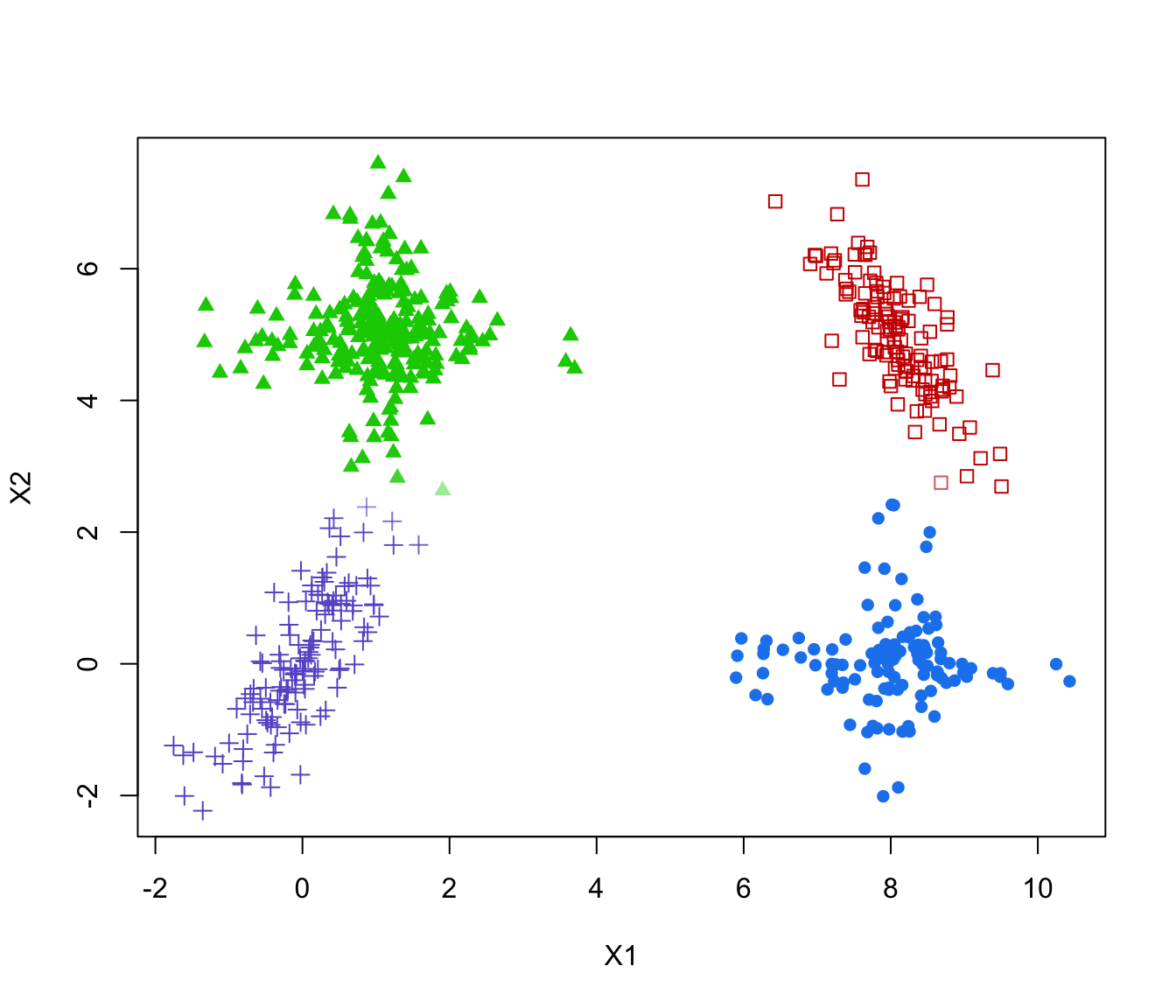Return the optimal number of clusters by combining mixture components based on the entropy method discussed in the reference given below.

clustCombiOptim(object, reg = 2, plot = FALSE, ...)

Arguments

object

An object of class 'clustCombi' resulting from a call to clustCombi.

reg

The number of parts of the piecewise linear regression for the entropy plots. Choose 2 for a two-segment piecewise linear regression model (i.e. 1 change-point), and 3 for a three-segment piecewise linear regression model (i.e. 3 change-points).

plot

Logical, if TRUE an entropy plot is also produced.

...

Further arguments passed to or from other methods.

Value

The function returns a list with the following components:

numClusters.combi

The estimated number of clusters.

z.combi

A matrix whose [i,k]th entry is the probability that observation i in the data belongs to the kth cluster.

cluster.combi

The clustering labels.

Author

J.-P. Baudry, A. E. Raftery, L. Scrucca

#>  $numClusters.combi: int 4 #>$ z.combi          : num [1:600, 1:4] 1.00 1.00 5.78e-04 1.85e-42 3.85e-35 ...
#>  $cluster.combi : num [1:600] 1 1 2 3 3 4 4 3 3 2 ... # plot optimal clustering with alpha color transparency proportional to uncertainty zmax <- apply(combiOptim$z.combi, 1, max)
col <- mclust.options("classPlotColors")[combiOptim$cluster.combi] vadjustcolor <- Vectorize(adjustcolor) alphacol = (zmax - 1/combiOptim$numClusters.combi)/(1-1/combiOptim$numClusters.combi) col <- vadjustcolor(col, alpha.f = alphacol) plot(ex4.1, col = col, pch = mclust.options("classPlotSymbols")[combiOptim$cluster.combi])